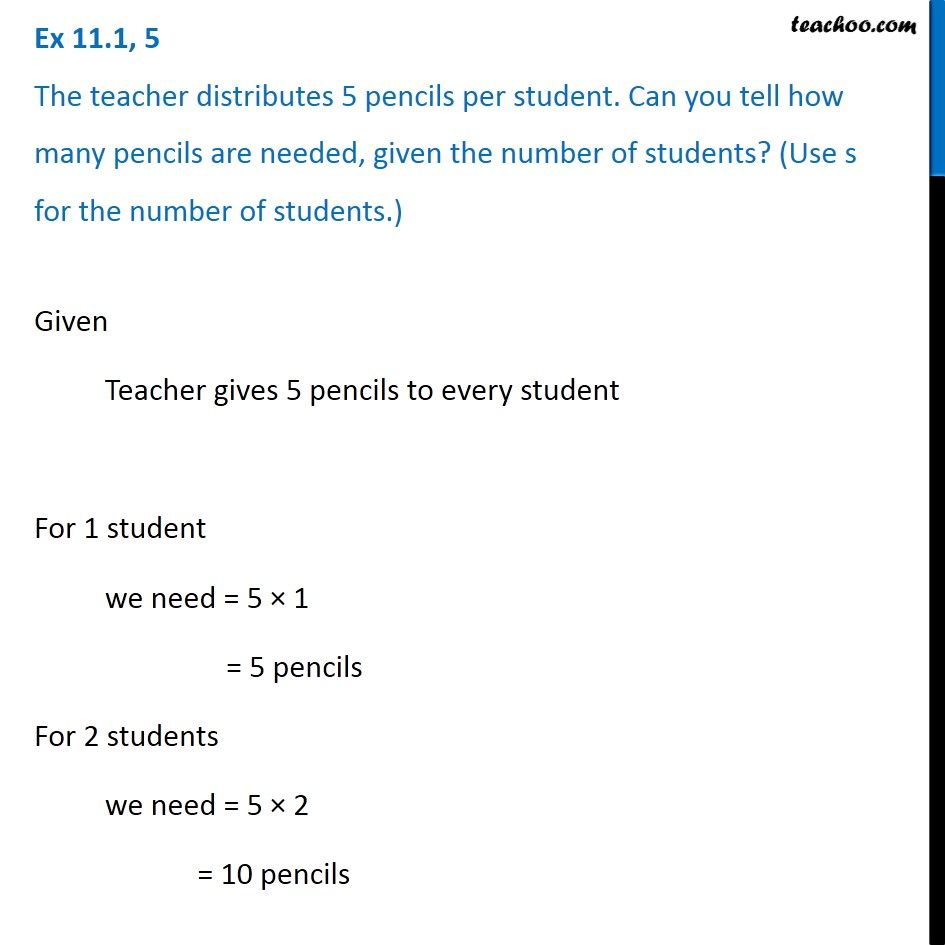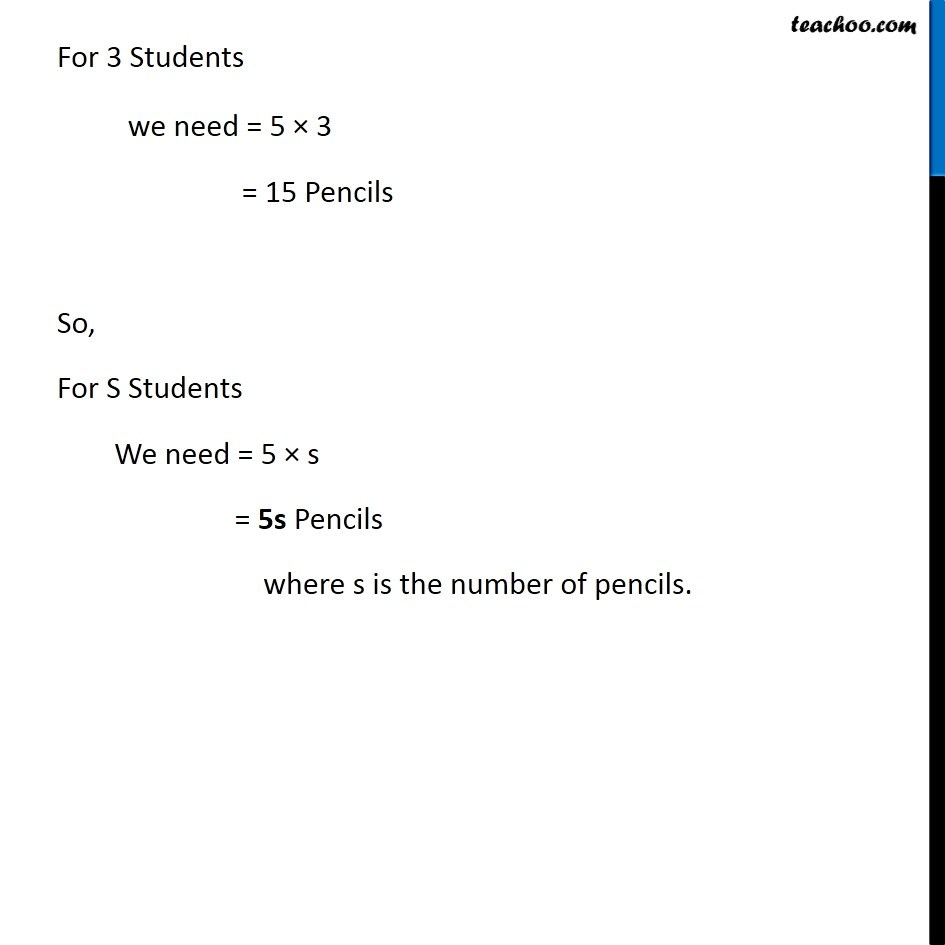Ex 11.1

Chapter 11 Class 6 Algebra
Serial order wiseGet live Maths 1-on-1 Classs - Class 6 to 12

### Transcript

Ex 11.1, 5 The teacher distributes 5 pencils per student. Can you tell how many pencils are needed, given the number of students? (Use s for the number of students.) Given Teacher gives 5 pencils to every student For 1 student we need = 5 × 1 = 5 pencils For 2 students we need = 5 × 2 = 10 pencils For 3 Students we need = 5 × 3 = 15 Pencils So, For S Students We need = 5 × s = 5s Pencils where s is the number of pencils.